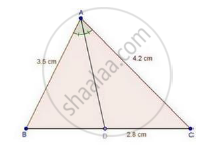Share

In a δAbc, Ad is the Bisector of ∠A, Meeting Side Bc at D. If Ab = 3.5 Cm, Ac = 4.2 Cm and Dc = 2.8 Cm, Find Bd. - CBSE Class 10 - Mathematics

Question

In a ΔABC, AD is the bisector of ∠A, meeting side BC at D.

If AB = 3.5 cm, AC = 4.2 cm and DC = 2.8 cm, find BD.

SolutionIn ΔABC, AD is the bisector of ∠A.

We know that, the internal bisector of an angle of a triangle divides the opposite side internally in the ratio of the sides containing the angle.

therefore"BD"/"DC"="AB"/"AC"

rArr"BD"/2.8=3.5/4.2

=(3.5xx2)/3

=7/3=2.33  cm

∴ 𝐵𝐷 = 2.3 cm

Is there an error in this question or solution?

Video TutorialsVIEW ALL 

Solution In a δAbc, Ad is the Bisector of ∠A, Meeting Side Bc at D. If Ab = 3.5 Cm, Ac = 4.2 Cm and Dc = 2.8 Cm, Find Bd. Concept: Angle Bisector.
S